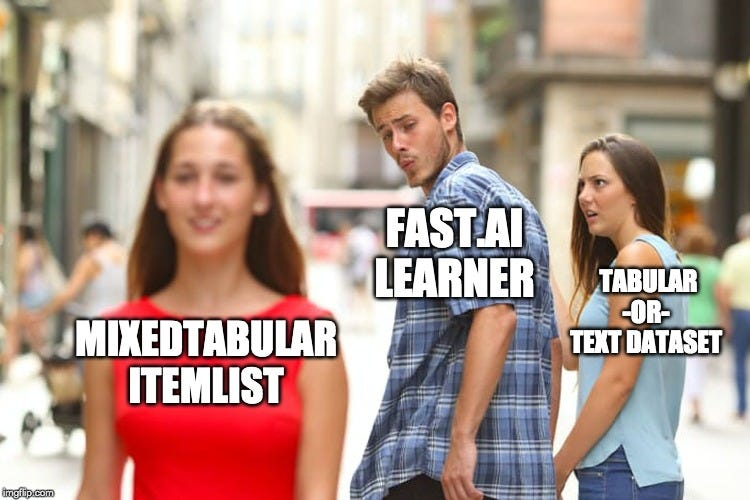# Finding Data Block Nirvana (a journey through the fastai data block API) — Part 2

Source: Deep Learning on Medium# Keep things DRY

I moved all the DataBlock API related code in the `utils.py` file which I then import all from at the top of the notebook. Since I’m likely to want to reuse this code elsewhere, it’s good to remember one of the golden maxims of programming: Don’t Repeat Yourself.

# Fixes to the DataBlock API bits

When I first attempted training my model I noticed that the tensors weren’t quite being grouped right in the `mixed_tabular_pad_collate` function. I fixed that in both the part 1 notebook and `utils.py` file, but I also left the old, wrong code, in the part 1 file so you can review what the output should, and should not, look like. I recommend you run the `yelp-00-custom-itemlist` notebook with both the corrected version or previous version to see the difference yourself.

# Fine-tuning the LM

Since we are working with text, I figured it made sense to actually fine-tune the AWD LSTM based ULMFit model using the target text available in our dataset. See the LM Fine-tuning section of the notebook. I illustrate the basic steps required to do this, and I’m sure its one of the many places where improvements can be made.

# Building the MixedTabular DataBunch

Remember all that code we wrote in part 1? Well, all that hard work has made it as simple as this to actually use it in our modeling task at hand.

`data_cls = (MixedTabularList.from_df( train_df, cat_cols, cont_cols, txt_cols, vocab=data_lm.train_ds.vocab,  procs=procs, path=PATH) .split_by_rand_pct(valid_pct=0.1, seed=42) .label_from_df(dep_var) .databunch(bs=32))`

This should look very familiar to anyone using the fast.ai framework. Notice how we are using the `vocab` from our fine-tuned language model above.

# TabularTextNN

How do we use this DataBunch? We’ll, I’m sure there are better ways than the one I present here, but I was able to get decent results from merely utilizing the models created from `tabular_learner` and `text_classifier_learner` fast.ai Learners. I definitely believe the approach is at least novel (at least I haven’t seen this anywhere) and can likely be improved upon.

As for the configuration required by both learners above, I decided to use a simply dictionary to make experimentation simple. See the respective `tabular_args` and `text_args` variables declared just above the definition of the `TabularTextNN` module.

The module’s `init` is where all the interesting things are:

`def __init__(self, data, tab_layers, tab_args={}, text_args={}): super().__init__() tab_learner = tabular_learner(data, tab_layers, **tab_args) tab_learner.model.layers = tab_learner.model.layers[:-1] self.tabular_model = tab_learner.model text_class_learner = text_classifier_learner(data, AWD_LSTM,  **text_args) text_class_learner.load_encoder('lm_ft_enc') self.text_enc_model = /  list(text_class_learner.model.children()) self.bn_concat = nn.BatchNorm1d(400*3+100) self.lin = nn.Linear(400*3+100, 50) self.final_lin = nn.Linear(50, data.c)`

If you look at the model returned by the tabular leaner here, you’ll see that the last layer is a linear that outputs the number of expected label the model needs to predict. As we’re going to be merging the outputs of this model with the text outputs before getting the probabilities for our labels, we just chop it off by setting the model layers equal to `tabular_learner.model.layers[:-1]`.

Similarly, we only need the text classification learner’s encoder for our purposes here, and so we remove the `PoolingClassifier` from it via `list(text_class_learner.model.children())`. Learning how to manipulate the PyTorch models as I have here is extremely helpful to understand and I’ve included a few resources below that were instructive for me.

The final step in our `forward()` function is to concatenate the results from both models, run them through a batch normalization layer and a couple of linear layers to get our predicted values. Notice how I also employ the concat pooling trick used in fast.ai to take advantage of all the information returned by the text encoder.

# Training

Guess what? You train this just like any other fast.ai model. That means there really isn’t anything new to learn here. You can just stick this model in a learner as such:

`model = TabularTextNN(data_cls, tab_layers, tabular_args, text_args)learn = Learner(data_cls, model, metrics=[accuracy])`

Nice, huh?